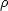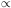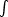### 2. SHELL EXPANSION

Expanding shells are most commonly made by stellar pressures in the form of HII regions, supernovae, and winds. If we write the expansion speed as dR / dt ~ (P /)1/2 for an isothermal shock, then the radius varies as a power law in time if the pressure P is a function of radius R and the densityis uniform. For an HII region, P = 2.1nkT where n = (3S / 4R3)1/2 for ionizing luminosity S in photons per second and recombination rateto all but the ground state. Then PR-3/2. For supernovae, P ~ 3E / 4R3 for the energy conserving, non-radiative, phase. For a wind, P ~ 3E(t) / 4R3, where the energy increases with time as E = Lt.

These three pressure-radius relations give three different radius-time expansion laws, dR / dtR-3/4 gives Rt4/7 for a Strömgren sphere, dR / dtR-3/2 gives R ~ t2/5 for the Sedov phase of a supernova, and dR / dtt1/2R-3/2 gives Rt3/5 for a steady wind or continuous energy supply from multiple supernovae in an OB association (Castor et al. 1975).

There are many complications to these solutions. External pressure is always present, slowing down the bubbles. External pressure Pext enters the expression as dR / dt = ([P - Pext] /)1/2 with Pext ~ constant. The solution is not a power law in this case. A second complication is the momentum in the moving shell. When this is important, the equation of expansion is really d(4R3 v/3) / dt = 4R2 (P - Pext). Shell momentum makes the shell move faster at a given radius than in the case without momentum. There are also diverse shock jump conditions depending on the importance of magnetic fields and the equation of state for the shocked gas, such as adiabatic or isothermal, or whether the full energy equation is used to determine the post-shock temperature.

We can see how important external pressure is to these solutions by finding the fraction of shells that are at a pressure significantly above the external value. As noted above, each source has solutions R(t) and P(R), which can be re-written into a solution for pressure versus time, P(t). Thus there is a relation for the volume as a function of pressure, V(P). For a constant rate n0 of making bubbles, n(P)dP = n0 dt. Therefore n(P)dt / dP. The volume filling factor is f(P) = n(P)V(P). Now we see that for HII regions, f(P)P-4.17; for winds, f(P)P-4.5, and for supernovae, f(P)P-5.2. For all of these, approximately, f(P)dPAP-4.5dP for some constant A. If all of the volume is filled, then 1 =f(P)dP, and the average pressure is related to the minimum pressure as Pave = 1.4Pmin, which means f(P) = 1.15(P / Pave)-4.5/Pave. Thus, the probability that any of these regions has a pressure exceeding 10 times the average, f(P > 10Pave), is 0.31 × 0.13.5 ~ 10-4; similarly, f(P > 2Pave) ~ 0.03. Evidently, most pressure bursts from HII regions, winds and supernovae are within twice the average ISM pressure for most of their lives. Therefore the external pressure is important for them. Kim, Balsara & Mac Low (2001) ran numerical simulations of the ISM and found that most of the time, the pressure stayed within a factor of 2 of the average value.

The probability distribution function for pressure also suggests that the largest pressure bursts are close-range and short-lived. Thus significant over-pressures from stellar sources are most likely to occur close to those stars, as in an adjacent cloud. Most of the giant IR and HI shells discussed above are drifting by momentum conservation.Figure 2. A pillar in IC 1396 viewed at 8 µm with the Spitzer Space Telescope. Class I sources (the youngest) are identified by diamond shapes. There are three at the front of the head, one near the back part of the head, two on the lower part of the pillar and another in a shelf nearby (from Reach et al. 2009).